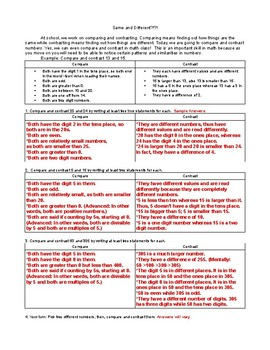# Compare and Contrast Numbers Using Place ValueSubject
Resource Type
File Type
PDF (69 KB|2 pages)
Standards
\$0.96
• Product Description
• Standards
This worksheet practices comparing and contrasting numbers, which will help later on when finding patterns, eliminating choices on standardized tests, and in algebra. It also allows a chance for students to write in math and to "construct viable arguments". While it does not require any of your typical calculations, it does require that students know and understand certain math vocabulary words, including place value.
Look for and make use of structure. Mathematically proficient students look closely to discern a pattern or structure. Young students, for example, might notice that three and seven more is the same amount as seven and three more, or they may sort a collection of shapes according to how many sides the shapes have. Later, students will see 7 × 8 equals the well remembered 7 × 5 + 7 × 3, in preparation for learning about the distributive property. In the expression 𝑥² + 9𝑥 + 14, older students can see the 14 as 2 × 7 and the 9 as 2 + 7. They recognize the significance of an existing line in a geometric figure and can use the strategy of drawing an auxiliary line for solving problems. They also can step back for an overview and shift perspective. They can see complicated things, such as some algebraic expressions, as single objects or as being composed of several objects. For example, they can see 5 – 3(𝑥 – 𝑦)² as 5 minus a positive number times a square and use that to realize that its value cannot be more than 5 for any real numbers 𝑥 and 𝑦.
Generate a number or shape pattern that follows a given rule. Identify apparent features of the pattern that were not explicit in the rule itself. For example, given the rule “Add 3” and the starting number 1, generate terms in the resulting sequence and observe that the terms appear to alternate between odd and even numbers. Explain informally why the numbers will continue to alternate in this way.
Compare two three-digit numbers based on meanings of the hundreds, tens, and ones digits, using >, =, and < symbols to record the results of comparisons.
Understand that the three digits of a three-digit number represent amounts of hundreds, tens, and ones; e.g., 706 equals 7 hundreds, 0 tens, and 6 ones. Understand the following as special cases:
Compare two two-digit numbers based on meanings of the tens and ones digits, recording the results of comparisons with the symbols >, =, and <.
Total Pages
2 pages